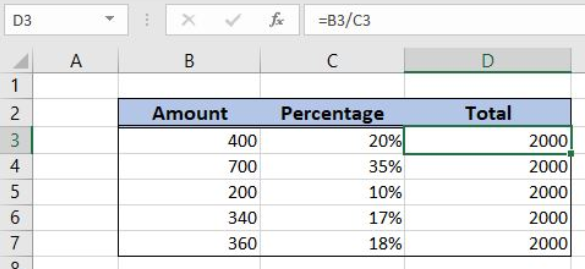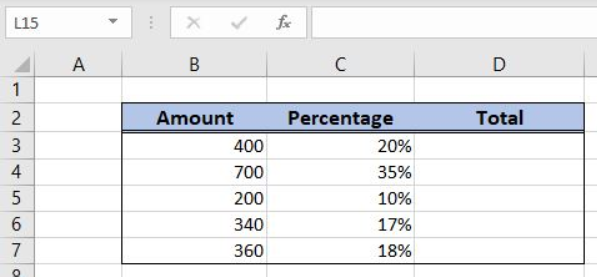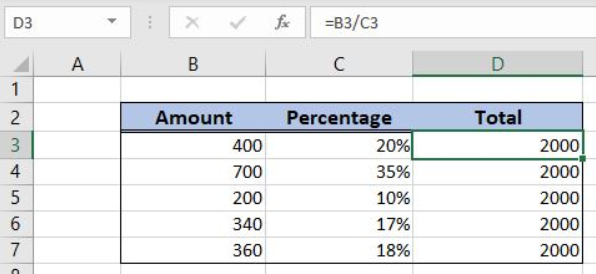Get instant live expert help with Excel or Google Sheets“My Excelchat expert helped me in less than 20 minutes, saving me what would have been 5 hours of work!”

#### Post your problem and you'll get expert help in seconds

Your message must be at least 40 characters
Our professional experts are available now. Your privacy is guaranteed.

# Get total from percentageRead time: 15 minutes

Excel allows a user to get a total from a percentage using the simple division. This step by step tutorial will assist all levels of Excel users in getting a total from a percentage.Figure 1. The result of the formula

## Syntax of the Formula

The generic formula is:

`=amount / percentage`

The parameters of the formula are:

• amount – an amount, representing the part of the total
• percentage – a percentage of the total.

## Setting up Our Data for the Formula

Let’s look at the structure of the data we will use. In column B (“Amount”), we have an amount and in column C (“Percentage”) we have a percentage. In the column D (“Total”), we want to get a total.Figure 2. Data that we will use in the example

## Get a Total from a Percentage

In our example, we want to get a total if 20% is 400. The result will be in the cell D3.

The formula looks like:

`=B3/C3`

The parameter amount is the cell B3, while the parameter percentage is C3.

To apply the formula, we need to follow these steps:

• Select cell D3 and click on it
• Insert the formula: `=B3/C3`
• Press enter
• Drag the formula down to the other cells in the column by clicking and dragging the little “+” icon at the bottom-right of the cell.Figure 3. Using the formula to get a total from a percentage

Finally, the result in the cell D3 is 2000.

Most of the time, the problem you will need to solve will be more complex than a simple application of a formula or function. If you want to save hours of research and frustration, try our live Excelchat service! Our Excel Experts are available 24/7 to answer any Excel question you may have. We guarantee a connection within 30 seconds and a customized solution within 20 minutes.

### Did this post not answer your question? Get a solution from connecting with the expert.Another blog reader asked this question today on Excelchat:## Subscribe to Excelchat.coAnother blog reader asked this question today on Excelchat: